IF YOU CAN SEE THIS go to /sfa/Nuprl/Shared/Xindentation_hack_doc.html

### Type Expressions

In Nuprl, as in Martin-Lof's 1980 type system, our expressions for Types are taken from the same general syntax of Expressions used for other values in the theory. This means that type expressions may be freely mixed with others. When one defines a type, one also specifies an expression to denote it. We sometimes use "A =ext B" to express equality between extensional types, i.e. for type expressions A and B, "A =ext B" holds when they denote the same partial equivalence relation. That is, A =ext B iff for all terms r and s, r = s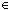A iff r = sB. See also the Subtype Relation.

Midway through Types some syntactic and computational restrictions are given for membership of, and equality between, expressions in a type, involving symmetry and transitivity as well. The same restrictions apply to type expressions themselves, and equality between type expressions (whether we are discussing extensional or Intensional Types).

The type expression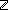is introduced as simply the class of integer literals, along with whatever terms evaluate to them.

But by far, the most common type definitions explain large classes of types given in terms of "constituent" types; these methods for specifying types are called "type constructors". For example, we stipulate that given a type A, there is a type "A List" whose members represent lists of members of type A, as described in Lists. Naturally, our notation "A List" for a list type has as a component a notation "A" for its constituent type.

We use the notation "A+B" for a Disjoint Union type when "A" and "B" denote types. This is a type constructor using two constituent types.

But it is also possible to define type constructors from a possibly infinite family of constituent types. For example, the type u:A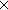B(u) is a type of pairs, as explained in Pairs, given any type A and any family of types B(x) (for xA). (It would be displayed as "AB" when B doesn't mention the binding variable.) One constituent type of this construction would be A, but also for each xA, B(x) is a constituent. Notationally, "u:AB(u)" is a binding operator, in which the variable "u" binds any free occurrences "B(u)".

Here is an example of a type with infinitely many constituent types.

Consider this type-valued function A^n designed to represent n-tuples of A's (see Recursive Functions)

A^0 *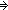Unit
A^1 *A
A^2 *AA
A^3 *AAA
et cetera.

The type "n: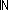A^n" has as its constituent typesand A^n for each n. This type represents all the finite tuples of A's each tagged with its tuple length.

The relation between a type and its constituent types is implicitly intended to be well-founded, i.e., the procedure of iteratively choosing a constituent type must always end.

(Sept 2001 - sfa )